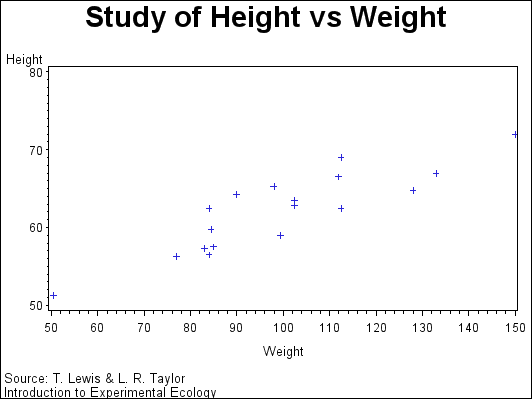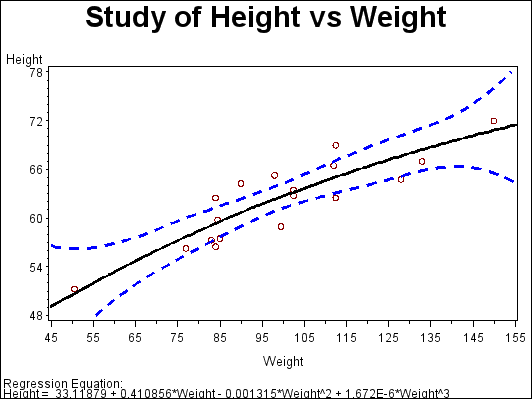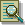The GPLOT Procedure

# Example 4: Plotting Two Variables

Procedure features:

PLOT statement options:

 HAXIS= HMINOR= REGEQN VAXIS=
Other features:

GOPTIONS statement option:

 BORDER

SYMBOL statement

Sample library member: GPLVRBL1In this example, the PLOT statement uses a plot request of the type y-variable*x-variable to plot the variable HEIGHT against the variable WEIGHT. The plot shows that weight generally increases with size.

This example then requests the same plot with some modifications. As shown by the following output, the second plot request specifies a regression analysis with confidence limits, and scales the range of values along the vertical and horizontal axes. It also displays the regression equation specified for the SYMBOL statement. Because the procedure supports RUN-group processing, you do not have to repeat the PROC GPLOT statement to generate the second plot.`goptions reset=all border;````title "Study of Height vs Weight"; footnote1 j=l "Source: T. Lewis & L. R. Taylor"; footnote2 j=l "Introduction to Experimental Ecology" j=r "GPLVRBL1(a) ";``````proc gplot data=sashelp.class; plot height*weight; run;````footnote1; /* this clears footnote1 */````symbol1 interpol=rcclm95 value=circle cv=darkred ci=black co=blue width=2;`````` plot height*weight / haxis=45 to 155 by 10 vaxis=48 to 78 by 6 hminor=1 regeqn; run; quit;```Previous Page | Next Page | Top of Page